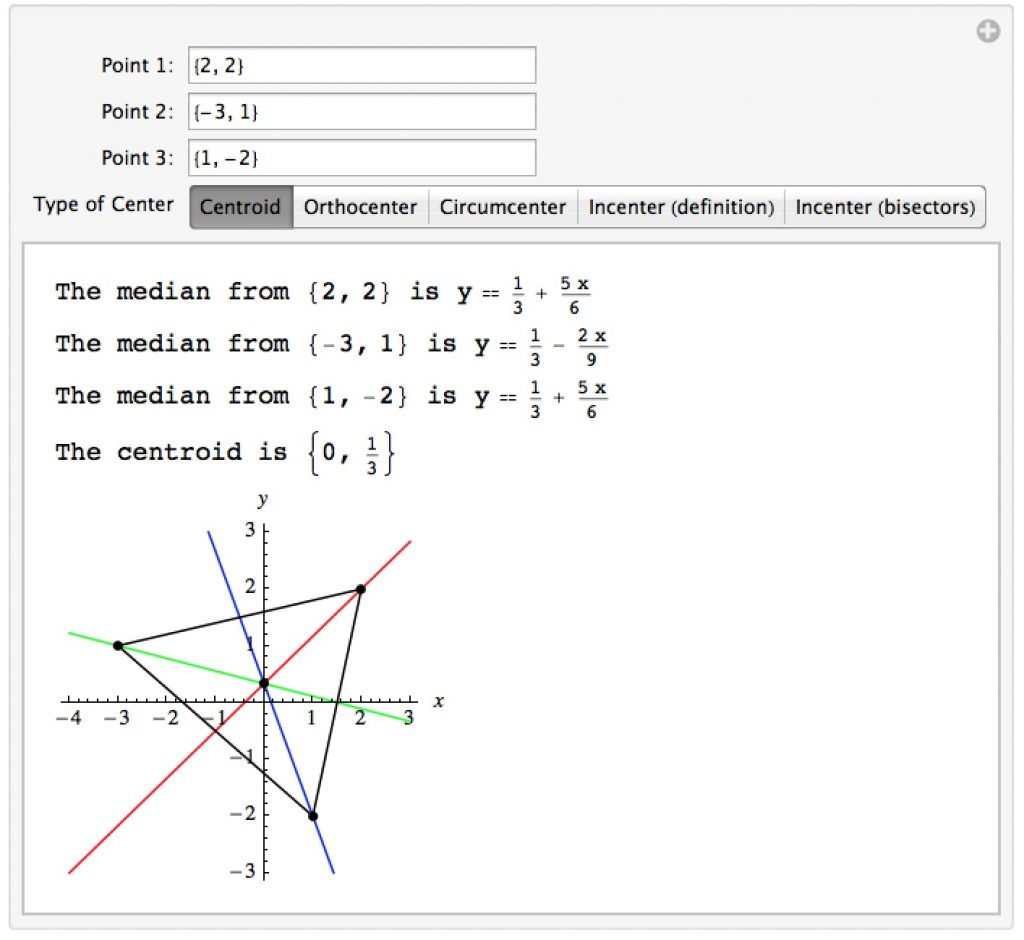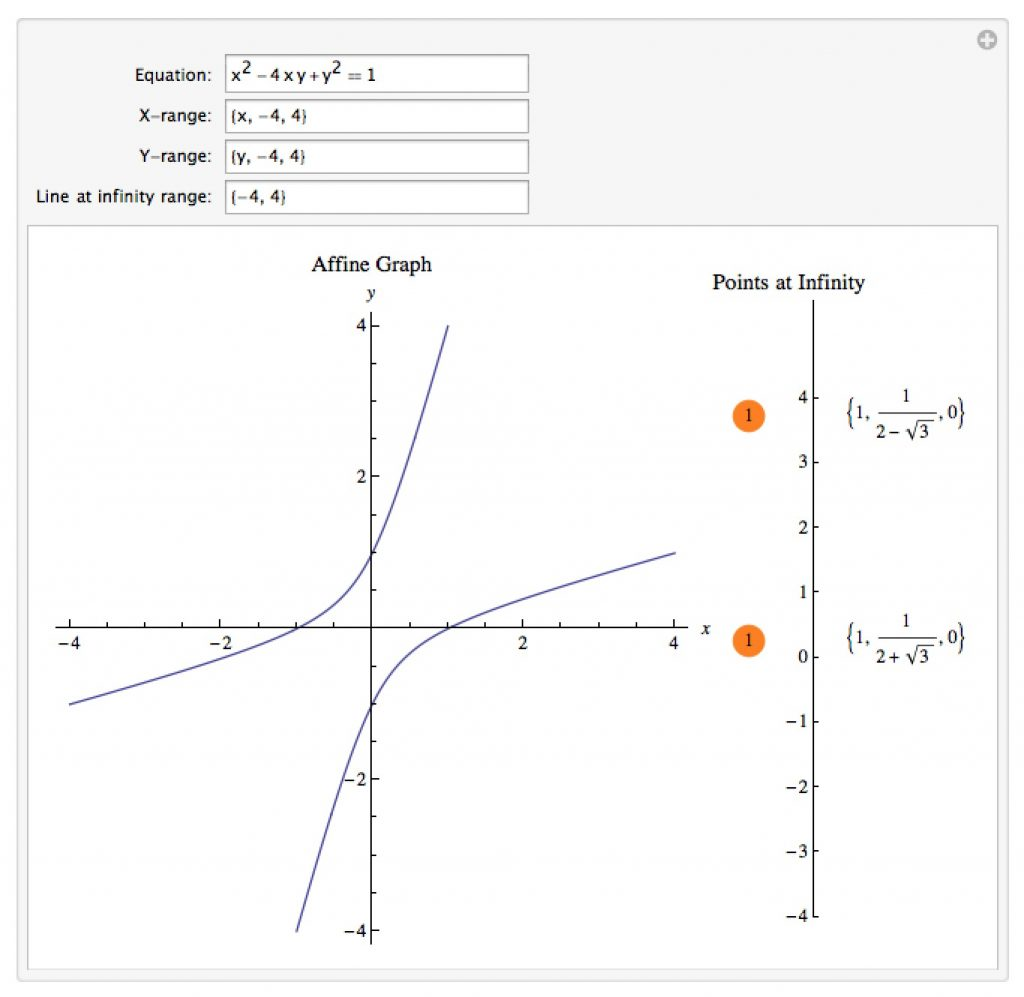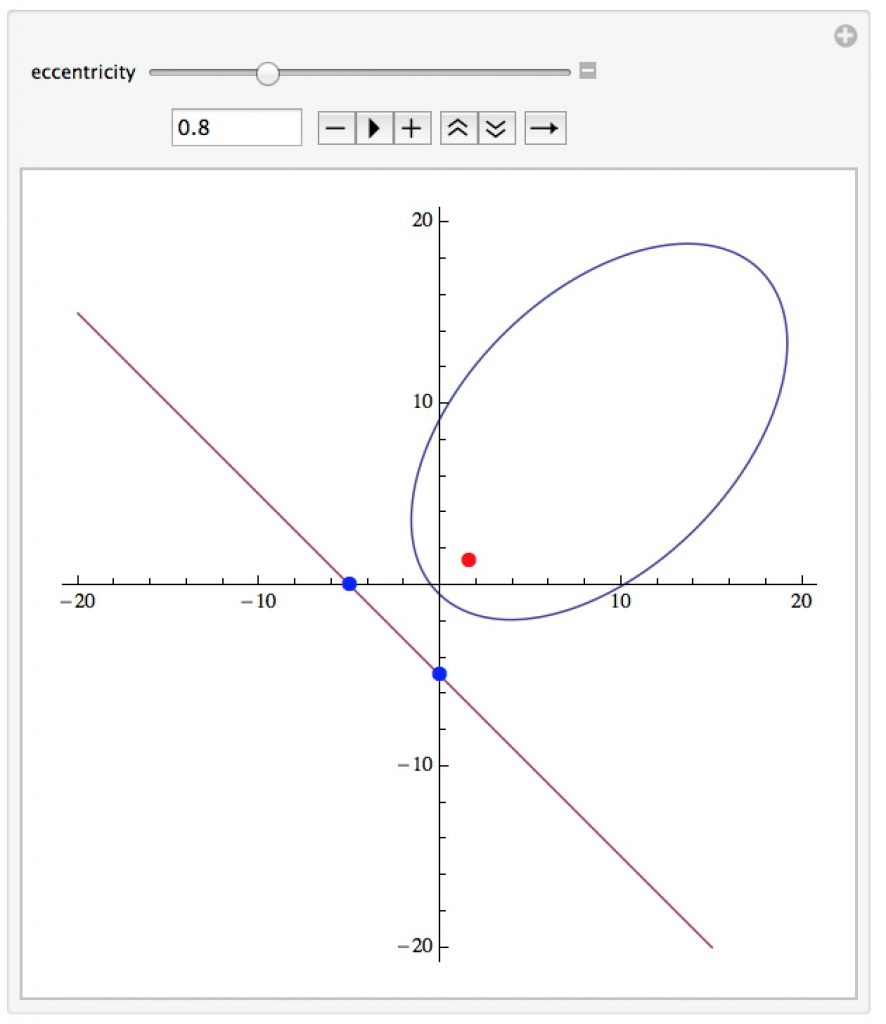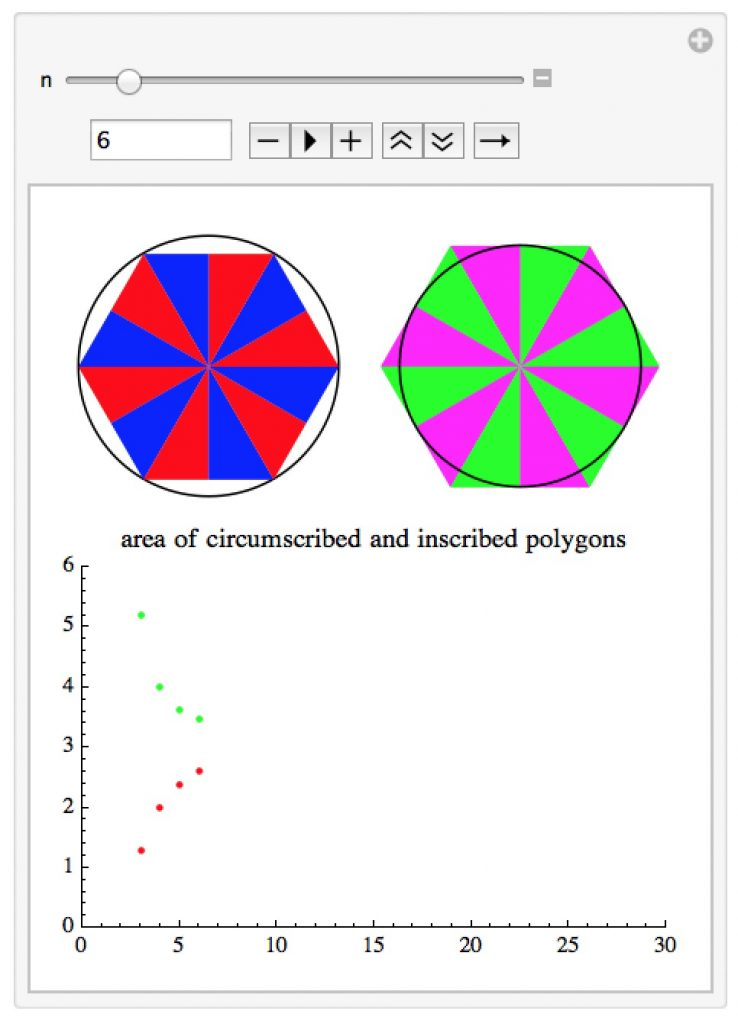# Mathematica Notebooks for Geometry

Important Note: The links for the notebooks open a new window or tab with a Google Drive page – the current settings for our homepages won’t allow me to host mathematica notebooks locally.

## Triangle Centers

This notebook lets you explore different notions of the center of a triangle – the centroid, circumcenter, and so on.## Projective Graphing

This notebook lets you graph an affine curve and find the “points at infinity” that correspond to the projective completion (and their multiplicity).## Circles Tangent to 3 Lines

This manipulation finds the circles which are tangent to 3 given lines.  Most of the time the equations of the circles will be fairly ugly even if the equations of the lines themselves are nice (the algebra underlying the problem involves solving a system of quadratic equations).## Conic Sections

This manipulation lets you experiment with the focus-directrix definition of a conic section.  You can move the focus, the points defining the directrix, and the eccentricity of the conic.